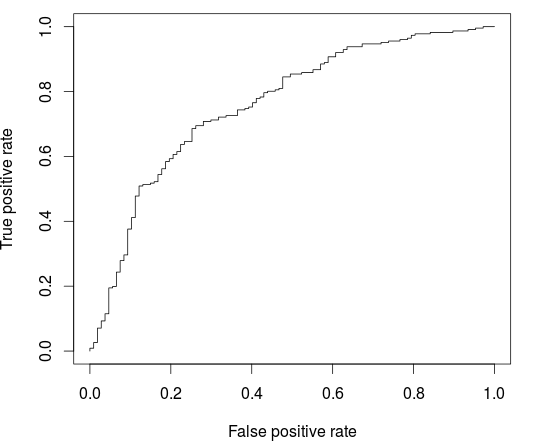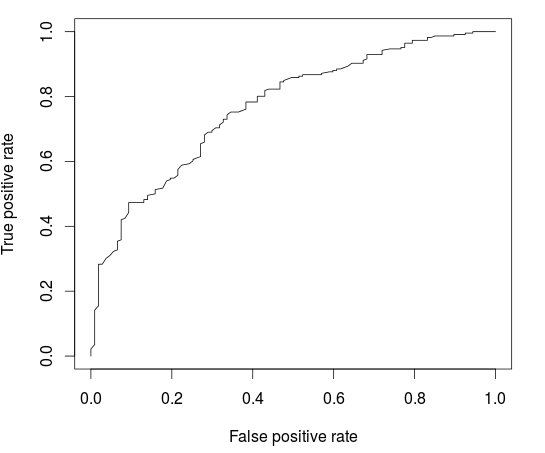Want to share your content on R-bloggers? click here if you have a blog, or here if you don't.

In our data science course, this morning, we’ve use random forrest to improve prediction on the German Credit Dataset. The dataset is

```> url="http://freakonometrics.free.fr/german_credit.csv"

Almost all variables are treated a numeric, but actually, most of them are factors,

```> str(credit)
'data.frame':	1000 obs. of  21 variables:
\$ Creditability   : int  1 1 1 1 1 1 1 1 1 1 ...
\$ Account.Balance : int  1 1 2 1 1 1 1 1 4 2 ...
\$ Duration        : int  18 9 12 12 12 10 8  ...
\$ Purpose         : int  2 0 9 0 0 0 0 0 3 3 ...```

(etc). Let us convert categorical variables as factors,

```> F=c(1,2,4,5,7,8,9,10,11,12,13,15,16,17,18,19,20)
> for(i in F) credit[,i]=as.factor(credit[,i])```

Let us now create our training/calibration and validation/testing datasets, with proportion 1/3-2/3

```> i_test=sample(1:nrow(credit),size=333)
> i_calibration=(1:nrow(credit))[-i_test]```

The first model we can fit is a logistic regression, on selected covariates

```> LogisticModel <- glm(Creditability ~ Account.Balance + Payment.Status.of.Previous.Credit + Purpose +
Length.of.current.employment +
Sex...Marital.Status, family=binomial,
data = credit[i_calibration,])```

Based on that model, it is possible to draw the ROC curve, and to compute the AUC (on ne validation dataset)

```> fitLog <- predict(LogisticModel,type="response",
+                   newdata=credit[i_test,])
> library(ROCR)
> pred = prediction( fitLog, credit\$Creditability[i_test])
> perf <- performance(pred, "tpr", "fpr")
> plot(perf)
> AUCLog1=performance(pred, measure = "auc")@y.values[]
> cat("AUC: ",AUCLog1,"n")
AUC:  0.7340997```An alternative is to consider a logistic regression on all explanatory variables

```> LogisticModel <- glm(Creditability ~ .,
+  family=binomial,
+  data = credit[i_calibration,])```

We might overfit, here, and we should observe that on the ROC curve

```> fitLog <- predict(LogisticModel,type="response",
+                   newdata=credit[i_test,])
> pred = prediction( fitLog, credit\$Creditability[i_test])
> perf <- performance(pred, "tpr", "fpr")
> plot(perf)
> AUCLog2=performance(pred, measure = "auc")@y.values[]
> cat("AUC: ",AUCLog2,"n")
AUC:  0.7609792```There is a slight improvement here,  compared with the previous model, where only five explanatory variables were considered.

Consider now some regression tree (on all covariates)

```> library(rpart)
> ArbreModel <- rpart(Creditability ~ .,
+  data = credit[i_calibration,])```

We can visualize the tree using

```> library(rpart.plot)
> prp(ArbreModel,type=2,extra=1)```The ROC curve for that model is

```> fitArbre <- predict(ArbreModel,
+                     newdata=credit[i_test,],
+                     type="prob")[,2]
> pred = prediction( fitArbre, credit\$Creditability[i_test])
> perf <- performance(pred, "tpr", "fpr")
> plot(perf)
> AUCArbre=performance(pred, measure = "auc")@y.values[]
> cat("AUC: ",AUCArbre,"n")
AUC:  0.7100323```As expected, a single has a lower performance, compared with a logistic regression. And a natural idea is to grow several trees using some boostrap procedure, and then to agregate those predictions.

```> library(randomForest)
> RF <- randomForest(Creditability ~ .,
+ data = credit[i_calibration,])
> fitForet <- predict(RF,
+                     newdata=credit[i_test,],
+                     type="prob")[,2]
> pred = prediction( fitForet, credit\$Creditability[i_test])
> perf <- performance(pred, "tpr", "fpr")
> plot(perf)
> AUCRF=performance(pred, measure = "auc")@y.values[]
> cat("AUC: ",AUCRF,"n")
AUC:  0.7682367```Here this model is (slightly) better than the logistic regression. Actually, if we create many training/validation samples, and compare the AUC, we can observe that – on average – random forests perform better than logistic regressions,

```> AUC=function(i){
+   set.seed(i)
+   i_test=sample(1:nrow(credit),size=333)
+   i_calibration=(1:nrow(credit))[-i_test]
+   LogisticModel <- glm(Creditability ~ .,
+    family=binomial,
+    data = credit[i_calibration,])
+   summary(LogisticModel)
+   fitLog <- predict(LogisticModel,type="response",
+                     newdata=credit[i_test,])
+   library(ROCR)
+   pred = prediction( fitLog, credit\$Creditability[i_test])
+   AUCLog2=performance(pred, measure = "auc")@y.values[]
+   RF <- randomForest(Creditability ~ .,
+   data = credit[i_calibration,])
+   fitForet <- predict(RF,
+                       newdata=credit[i_test,],
+                       type="prob")[,2]
+   pred = prediction( fitForet, credit\$Creditability[i_test])
+   AUCRF=performance(pred, measure = "auc")@y.values[]
+   return(c(AUCLog2,AUCRF))
+ }
> A=Vectorize(AUC)(1:200)
> plot(t(A))```